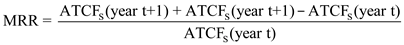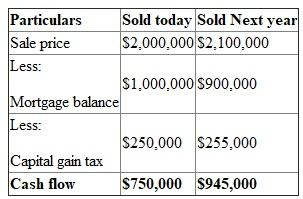## Quiz 14 : Disposition and Renovation of Income PropertiesLooking for Finance Homework Help?# Quiz 14 : Disposition and Renovation of Income Properties

The Marginal Rate of Return (MRR) is calculated to get the rate of return the investor will get by selling the property today or by keeping the property for an additional year or more years. It basically refers to the rate of return an investor will get by holding the property for one additional year. The formula to calculate Marginal Rate of Return is as follows:Here, ATCF s is After-tax cash flow from sale t is Number of years A property could be sold for \$2,000,000. Loan balance is \$1,000,000. Capital gain tax if sold is \$250,000. If property sold today then Internal Rate of Return (IRR) is 15%. If property is not sold then after-tax cash flow or net operating income for next year will be \$50,000. At the end of the year, property value will increase and be \$2,100,000. Loan balance will decrease to \$900,000. Capital gain due will increase and be \$255,000. (a) Firstly lets calculate the cash flow if the property is sold today or the property is sold next year.Calculate the Marginal Rate of Return based on the formula as follows:Here, ATCF s is After-tax cash flow from sale NOI is Net operating income t is Number of years Therefore, Marginal Rate of Return (MRR) is 32.7%. (b) The investor should hold the property till next year. The MRR is higher than the IRR. This means that if the property will be hold it will yield higher return next year. The cash flow of \$750,000 can be invested at a higher rate i.e. MRR rather than at rate of IRR.

Income property: An income property can be defined as the property purchased or developed to earn income by various means, such as leasing, renting, or cost appreciation. Such property may be commercial or residential in nature. Residential income properties are also called non-owner occupied. The mortgage for a non-owner occupied property would hold a comparatively higher rate of interest as compared to a mortgage occupied by the owner. This is primarily because such mortgages are often considered to be of higher risk by the lenders. Factors to be considered by an investor when deciding whether to dispose a property that he has owned for many years: The factors to be considered by an investor when deciding whether to dispose a property that he has owned for many years are usually based on a set of criteria, such as incremental, marginal, or returns. The investor is required to analyze the anticipated performance of the property in the future before comparing the internal rate of return for holding versus sale of property. The investor should also analyze whether the net funds earned from a property sale can be reinvested at a higher return rate as compared to the return which would be obtained when the property is not sold. The investor is further required to analyze the taxation laws in effect while purchasing or selling a property. Any change in the tax law has an impact on the associated benefits of the current versus new investors in the same property.

Return from investment refers to the yield or return earned from an investment. The formula to calculate the return on investment for property renovation is as follows:If the property is renovated then after tax cash flow from operations is \$60,000 and if the property is not renovated then after tax cash flow from operations is \$50,000. This means that incremental cash flows from operations is \$10,000. If the property is renovated then sale proceeds from sale will be \$2,400,000 and If the property is not renovated then sale proceeds from sale will be \$2,100,000. This means that incremental cash flows from sale is \$300,000. (a) Calculate the return from renovation as shown follows:Therefore, the return from investment or rate of return is 24%. (b) Since the rate of return from renovation is 24% which is very high. Therefore, an investor can consider this option if there is no additional risk involved or \$750,000 will not give a higher rate of return if invested somewhere else.